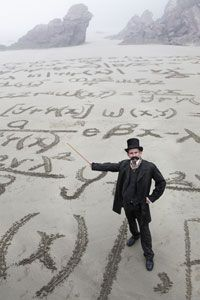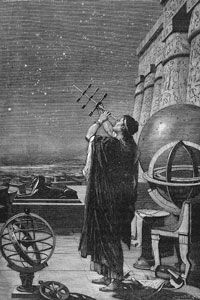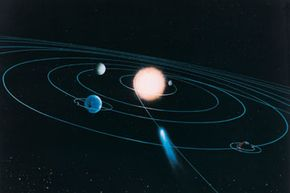How Math Works

By: Robert LambDon't fear the math.

It's easy to think of mathematics as a kind of storybook sorcery -- a powerful secret language known to few, mastered by inhuman agents (such as your calculator) and underpinning the very fabric of the universe. Even if we avoid such hyperbole, the fact remains: Many of us are mathematically illiterate in a world that runs on math.

When was the last time you seriously crunched some numbers with only pen and paper? In his book "The Geometry of Paradise," Mark A. Peterson described the people of medieval Europe as a nonmathematical culture in possession of sophisticated mathematics. Mathematicians of the day certainly honed their skills but mostly out of love for mathematical abstractions. They perused few practical applications with it and, according to Peterson, didn't really grasp what math was.

Today, the mathematics field is far more vibrant than it was in the Middle Ages, but it still eludes an alarming number of those who depend on it. On one hand, math certainly has a way of solving itself these days through calculators and hastily keyed-in Google searches. Yet for many individuals, mathematical anxiety begins with inadequate teaching from nonmathematicians who have trouble relaying enthusiasm and practicality. Factor in overcrowded classes, and it's little wonder that so many students fail to latch onto math's logical core. In fact, only 40 percent of 4th graders and 34 percent of 8th graders in the U.S. are proficient in math, according to Arne Duncan, U.S. education secretary speaking at the National Council of Teachers of Mathematics in April 2011.

The ramifications of mathematical illiteracy are very real. In 2005, the United States National Academies identified the country's decline in mathematics education as having a severe detrimental effect on its scientific, technological and economic prowess [source: Mullich].

So let's demystify the world of mathematics. A world without math is unimaginable. It's a part of who we are. It's the analytical juice of our left brain and, in the words of physicist Richard Feynman, even a fool can use it. Here's a quote from the late great scientist's book "The Pleasure of Finding Things Out":

What we've been able to work out about nature may look abstract and threatening to someone who hasn't studied it, but it was fools who did it, and in the next generation, all the fools will understand it. There's a tendency to pomposity in all this, to make it deep and profound.

In this article, we'll take a very wide-angle look at the world of numbers. Just what are they, and what does math really do?

What Are Numbers?A boxing referee administers the count.

Mathematics boils down to pattern recognition. We identify patterns in the world around us and use them to navigate its challenges. To do all this, however, we need numbers -- or at least the information that our numbers represent.

What are numbers? As we'll explore more later, that's a deceptively deep question, but you already know the simple answer. A number is a word and a symbol representing a count. Let's say you walk outside your home and you see two angry dogs. Even if you didn't know the word "two" or know what the corresponding numeral looks like, your brain would have a good grasp of how a two-dog encounter compares with a three-, one- or zero-dog situation.

We owe that innate comprehension to our brain (specifically, the inferior parietal lobe), which naturally extracts numbers from the surrounding environment in much the same way it identifies colors [source: Dehaene]. We call this number sense, and our brains come fully equipped with it from birth. Studies show that while infants have no grasp of human number systems, they can still identify changes in quantity.

Neuroimaging research has even discovered that infants possess the ability to engage in logarithmic counting, or counting based on integral increases in physical quantity. While a baby won't see the difference between five teddy bears and six teddy bears in a lineup, he or she will notice a difference between five and 10 [source: Miller].

Number sense plays a vital role in the way animals navigate their environments -- environments where objects are numerous and frequently mobile. However, an animal's numerical sense becomes more imprecise with increasingly larger numbers. Humans, for instance, are systematically slower to compute 4 + 5 than 2 + 3 [source: Dehaene].

At some point in our ancient past, prehistoric humans began to develop a means of augmenting their number sense. They started counting on their fingers and toes. This is why so many numerical systems depend on groups of five, 10 or 20. Base-10 or decimal systems stem from the use of both hands, while base-20 or vigesimal systems are based on the use of fingers and toes.

So ancient humans learned to externalize their number sense and, in doing so, they arguably created humanity's most important scientific achievement: mathematics.

The Tower of Math: Numbers

Numbers pose a difficulty for humans. Sure, some of us have more of a gift for math than others, but every one of us reaches a point in our mathematical education where things become hard. Learning your multiplication tables is difficult because the human brain never evolved to handle such advanced computations as 17 x 32 = 544. After a certain point, our mathematical education is largely an exercise in rejiggering ill-adapted brain circuits [source: Dehaene].

Number sense may come naturally to us, but mathematical literacy comes only with time. Likewise, humanity's use of mathematics has steadily grown over the ages. Like science itself, math isn't the product of one mind but rather a steady accumulation of knowledge throughout human history.

Think of math as a tower. Natural human height is finite, so if we're to reach higher into the air and see out farther across the landscape, we'll need to build something external to ourselves. Our mental abilities to understand math are equally finite, so we build a great tower of number systems and climb upward to the stars.

To break down the basic structure of this tower, let's first look at the raw materials. These are the basic types of numbers:

Integers: You probably know these as whole numbers, and they come in both positive and negative forms. Integers include the basic counting numbers (1-9), negative numbers (-1) and zero.

Rational numbers include integers but also encompass simple fractions that can be expressed as a ratio of two integers. For example, 0.5 is rational because we can also write it as 1/2.

Irrational numbers: These numbers can't be written as a ratio of two integers. Pi (the ratio of the circumference of a circle to its diameter) is a classic example, as it can't be written accurately as a ratio of two integers and has been calculated to trail off decimal points into the trillions.

Rational and irrational numbers both fall under the category of real numbers or complex numbers. And yes, there are also imaginary numbers that exist outside the real number line, and transcendental numbers, such as pi. There are many other different numbers types as well, and they, too, play a part in the structure of our tower.

On the next page, we'll look at some of the core branches of mathematics.

The Tower of Math: Branches of MathematicsCirca 100 B.C., Greek astronomer Hipparchus, inventor of trigonometry, studies the heavens.
Archive Photos/Getty Images

Who would you hire to build a tower? After all, several different systems converge in modern construction: steel framework, stone foundation, woodwork, plumbing, roofing, electrical wiring, telecommunications heating and air conditioning. Likewise, many branches of mathematics play a part in the tower of math. Here are just a few.

Arithmetic: This is the oldest and most basic form of mathematics. Arithmetic chiefly concerns the addition, subtraction, multiplication and division of real numbers that aren't negative.

Algebra: The next level of mathematics, algebra, is essentially arithmetic with unknown or abstract quantities thrown in with the real numbers. We represent the abstracts with symbols, such as X and Y.

Geometry: Remember what we said about math helping us navigate a world of numerous and movable objects? This is where geometry comes into play, dealing chiefly with the measurements and properties of points, lines, angles, surfaces and solids.

Trigonometry: Trigonometry concerns the measurements of triangles and the relationships between their sides and angles. While the historical origins of arithmetic, algebra and geometry are lost in the fog of ancient history, trigonometry originates with second century astronomer Hipparchus of Nicaea.

Calculus: Independently developed by both Isaac Newton and Gottfried Leibniz in the 17th century, calculus deals with the calculation of instantaneous rates of change (known as differential calculus) and the summation of infinite small factors to determine some whole (known as integral calculus). As such, it has proven a vital scientific tool in a number of disciplines.

The tower of mathematics has enabled human culture to rise and flourish, to understand both the inner mysteries of the cells to the outer mysteries of space.

But did we truly build this tower out of our own ingenuity? Did we invent mathematics or merely discover it? Explore this tantalizing question on the next page.

Math: Human Discovery or Human Invention?Does the universe conform to math, or math to the universe?
Digital Vision/Getty Images

So just what, in essence, is this thing called math? In developing these numbers and systems of numbers, did we discover the hidden coding of the universe? Is mathematics, in the words of Galileo, the language of God? Or is math just a human-created system that happens to correspond with natural laws and structures? There is no definitive answer to this question, but mathematicians tend to side with one of several compelling theories.

First, there is the Platonic theory. Greek philosopher Plato argued that math is a discoverable system that underlines the structure of the universe. In other words, the universe is made of math and the more we understand this vast interplay of numbers, the more we can understand nature itself. To put it more bluntly, mathematics exists independent of humans -- that it was here before we evolved and will continue on long after we're extinct.

The opposing argument, therefore, is that math is a man-made tool -- an abstraction free of time and space that merely corresponds with the universe. Just consider elliptical planetary orbits. While such an elliptical trajectory provides astronomers with a close approximation of the planet's movement, it's not a perfect one [source: Dehaene].

Several theories expand on this idea.

• The logistic theory, for instance, holds that math is an extension of human reasoning and logic.
• The intuitionist theory defines math as a system of purely mental constructs that are internally consistent.
• The formalist theory argues that mathematics boils down to the manipulation of man-made symbols. In other words, these theories propose that math is a kind of analogy that draws a line between concepts and real events.
• The fictionalist theory, while less popular, even goes so far as to equate mathematics with fairy tales: scientifically useful fictions. In other words, 1 + 1 = 2 might enable us to understand how the universe works, but it isn't a "true" statement.

Who's right? Who's wrong? There's ultimately no way to know, but on the next page we'll look at two examples of what each possibility could mean to our understanding of the universe.

The Mathematical UniverseCan math explain it all?
Image courtesy NASA/JPL-Caltech/R. Kennicutt/SINGS

The history of mathematics is a history of humanity seeking to understand the universe. Therefore, many consider the holy grail of mathematics to be the same as that of physics: a theory of everything, a unified theory that explains all physical reality.

Math generally plays a vital role in any theory of everything, but contemporary cosmologist Max Tegmark even goes so far as to theorize that the universe itself is made of math. In his mathematical universe hypothesis, he proposes that math is indeed a human discovery and that the universe is essentially one gigantic mathematical object. In other words, mathematics no more describes the universe than atoms describe the objects they compose; rather math is the universe. Tegmark even goes so far as to predict that a mathematical proof for a theory of everything could eventually fit on a T-shirt.

More than 60 years earlier, however, Austrian mathematician Kurt Gödel put forth a theory that argues quite the opposite. Gödel's first incompleteness theorem concerns axioms, logical mathematical statements that we assume to be true but can't be proven with a mathematical proof. A simple example of this would be the axiom of equality (X = X). We assume this to be a true statement, but we can't actually back it up with a mathematical proof. Gödel's theorem states that any adequate axiomatizable theory is incomplete or inconsistent.

The implication, according to theoretical physicist and mathematician Freeman Dyson, is that mathematics is inexhaustible. No matter how many problems we solve, we'll inevitably encounter more unsolvable problems within the existing rules [source: Feferman]. This would also seem to rule out the potential for a theory of everything, but it still doesn't relegate the world of numbers to either human invention or human discovery.

Regardless, mathematics could stand as humanity's greatest invention. It composes a vital part of our neural architecture and continues to empower us beyond the mental limits we were born with, even as we struggle to fathom its limits.

Explore the links on the next page to learn even more about mathematics.

Sources

• Cole, Marilyn. Personal interview. May 10, 2011.
• Courant, Richard and Herbert Robbins. "What is Mathematics?" Oxford University Press. July 18, 1996.
• Dehaene, Stanislas. "What Are Numbers, Really? A Cerebral Basis For Number Sense." Oct. 27, 1997. (April 19, 2011)http://www.edge.org/3rd_culture/dehaene/index.html
• Feferman, Solomon. "The nature and significance of Gödel's incompleteness theorems." Institute for Advanced Study, Princeton. Nov. 17, 2006. (April 25, 2011)http://math.stanford.edu/~feferman/papers/Godel-IAS.pdf
• Feynman, Richard P. "The Pleasure of Finding Things Out." Perseus Publishing. 1999.
• Hill, Josh. "Is Mathematics Discovered or Invented?" Daily Galaxy. April 28, 2008. (April 19, 2011)http://www.dailygalaxy.com/my_weblog/2008/04/is-mathematics.html
• Joyce, David E. "History of Trigonometry Outline." Clark University. (April 19, 2011)http://aleph0.clarku.edu/~djoyce/ma105/trighist.html
• Miller, Lulu. "Innate Numbers?" RadioLab. Nov. 30, 2009. (April 19, 2011)http://www.radiolab.org/2009/nov/30/innate-numbers/
• Mullich, Joe. "Rising to the Challenge." The Wall Street Journal. 2009. (April 21, 2011)http://online.wsj.com/ad/article/mathscience-rising
• Myers, Dale. "Godel's Incompleteness Theorem." Pacific Union College. (April 25, 2011)http://www.math.hawaii.edu/~dale/godel/godel.html#FirstIncompleteness
• Peterson, Mark A. "The Geometry of Paradise." The Mathematical Intelligencer. 2008.
• "Philosophy of Mathematics." Stanford Encyclopedia of Mathematics." Sept. 25, 2007.http://plato.stanford.edu/entries/philosophy-mathematics/
• Richardson, William H. "A Time-line for the History of Mathematics." Wichita State University. (April 19, 2011)http://www.math.wichita.edu/~richardson/
• Rosen, Gideon. "What are numbers?" Philosophy Talk. March 14, 2006. (April 19, 2011)http://www.philosophytalk.org/pastShows/Number.html
• Ross, Marty. "The Decline and Continued Fall of Australian Mathematics." Maths Masters. (May 10, 2011)http://www.qedcat.com/marty_ross_fall_of_mathematics.pdf
• Slavin, Steve. "All the Math You'll Ever Need." John Wiley & Sons, Inc. 1989.
• Tegmark, Mark. "Shut up and calculate." New Scientist. Sept.15, 2007. (April 25, 2011)http://arxiv.org/PS_cache/arxiv/pdf/0709/0709.4024v1.pdf
• U.S. Department of Education. "Math Teachers: The Nation Builders of the 21st Century: Remarks to the National Council of Teachers of Mathematics." Arne Duncan. April 15, 2011. (April 26, 2011)http://www.ed.gov/news/speeches/math-teachers-nation-builders-21st-century
• Way, Rupert. Personal interview. April 27, 2011.